Get instant live expert help with Excel or Google Sheets“My Excelchat expert helped me in less than 20 minutes, saving me what would have been 5 hours of work!”

#### Post your problem and you'll get expert help in seconds

Your message must be at least 40 characters
Our professional experts are available now. Your privacy is guaranteed.

# How to do Dynamic Reference of Table Name

With the INDIRECT function in combination with functions like SUM and AVERAGE, we can do dynamic reference of table name to perform different calculations. This tutorial will walk through the steps to dynamically reference a set of data in other functions.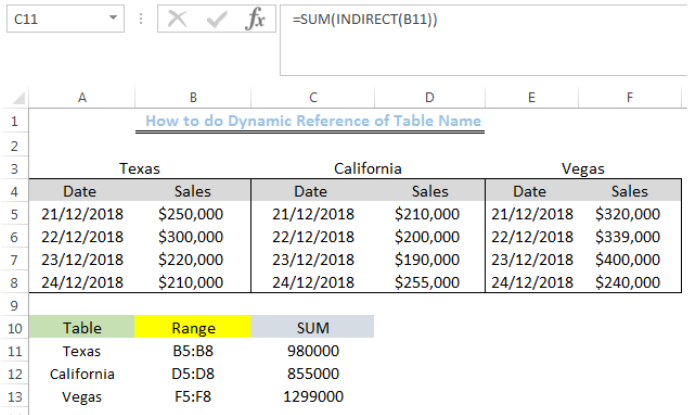Figure 1: Total Sales for the Three Cities

## Setting up the Data

• We will set up the data by inserting the dates and sales into Column A to Column F as shown in figure 2. We will also name them with the three cities as shown in the figure.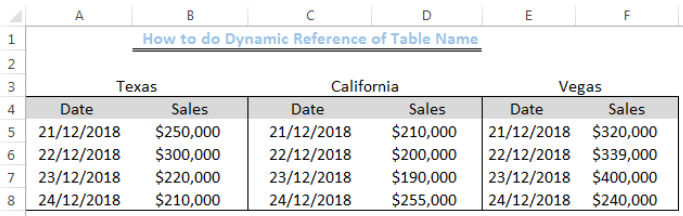Figure 2: Data showing three tables named “Texas,” “California” and “Vegas.”

## Dynamic Reference of Table Name with Range

We will input the cities into Cell A11 to Cell A13 and place the range of the sales in Cell B11 to Cell B13 as shown in figure 3.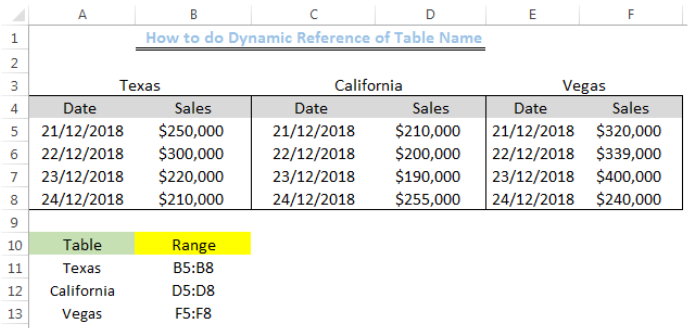Figure 3: Assigning selected range to each table name

## Syntax

`=function(INDIRECT(ref_text)`

## Formula

`=SUM(INDIRECT(B11)`

## Using the SUM and INDIRECT function

We will sum the sales of the different cities using the formula above. We will insert this formula into Cell C11.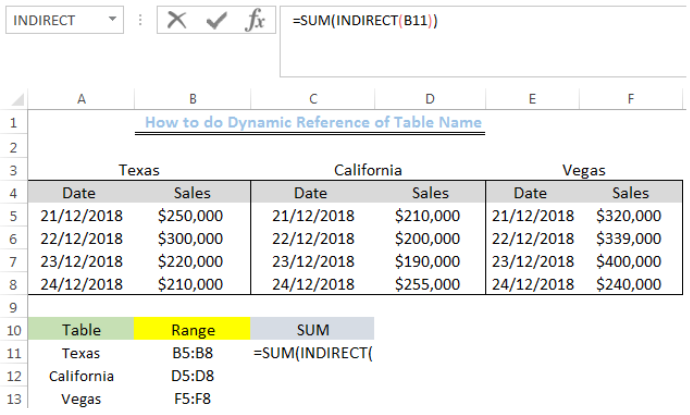Figure 4: Using the SUM function for Dynamic Reference of Table Name

We will press enter to get the total sales.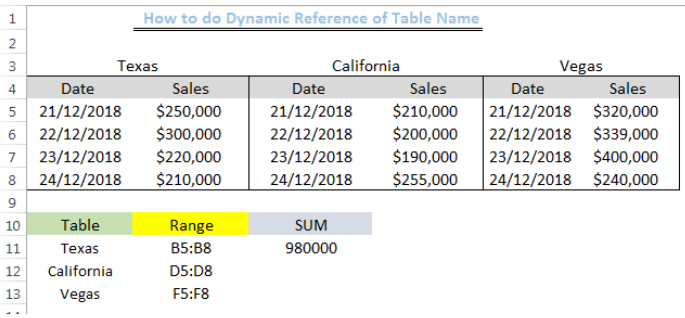Figure 5: Total Sales for Texas

We will use the drop-down to get the total sales for California and Vegas.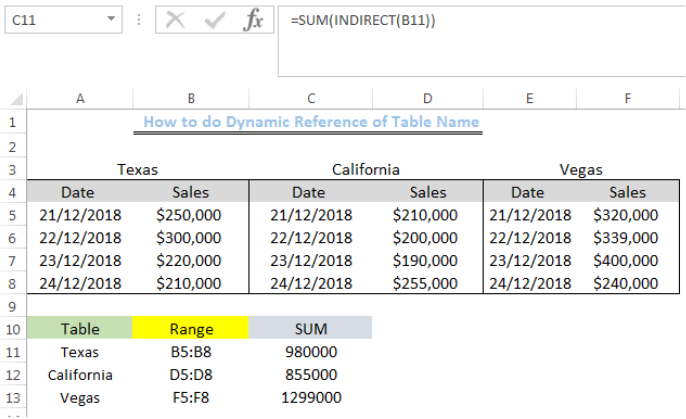Figure 6: Total Sales for the Three Cities

## Note

We can find the average sales by inputting the formula below:

`=AVERAGE(INDIRECT(B11))`

We can use other combinations with the dynamic reference to the table name.

## Explanation

The INDIRECT function creates a dynamic reference to the table name “Texas” and returns the values of the Range for the table name, which, in this case, is “B5:B8”.  The SUM FUNCTION then totals the values in Cells B5 to B8.

## Instant Connection to an Expert through our Excelchat Service

Most of the time, the problem you will need to solve will be more complex than a simple application of a formula or function. If you want to save hours of research and frustration, try our live Excelchat service! Our Excel Experts are available 24/7 to answer any Excel question you may have. We guarantee a connection within 30 seconds and a customized solution within 20 minutes.

### Did this post not answer your question? Get a solution from connecting with the expert.Another blog reader asked this question today on Excelchat:## Subscribe to Excelchat.coAnother blog reader asked this question today on Excelchat: